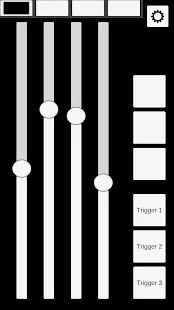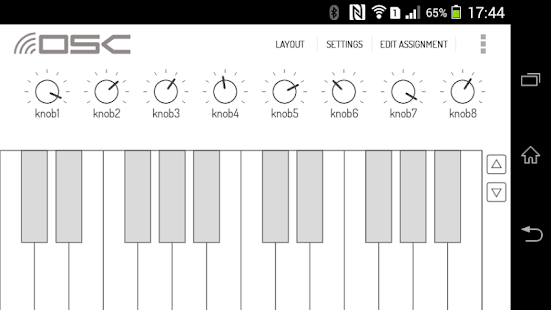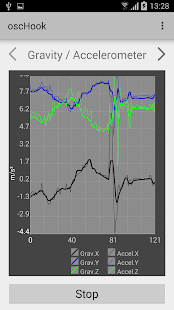# Free OCS Android app

Simply enter the IP address of the sonic-pi host in the android application settings and allow OSC messages in sonic-pi.

## OSC ControllerExample: Play a sample on sonic-pi by an OSC Controller button and control the amplitude with an OSC Controller slider.

``````live_loop :foo do
a = sync "/osc/oscControl/button1"
b = get "/osc/oscControl/slider1"
if  a == 1
sample :bd_boom, amp: b
end
end
``````

## OSC SurfaceExample: Play a synth on sonic-pi using an OSC Surface keyboard and control the amplitude with an OSC Surface slider(knob1).

``````live_loop :foo do
a = sync "/osc/note"
b = get "/osc/cc/1"
if a>0
synth :beep, note: a,amp: b
end
end
``````

## OscHookExample: Control the ocean wave in Sonic-pi with the sensors of an Android smartphone.

``````with_fx :reverb, mix: 0.5 do
live_loop :foo do
a = sync "/osc/accelerometer/raw/y"
if a>7
s = synth [:bnoise, :cnoise, :gnoise].choose, amp: rrand(0.5, 1.5), attack: rrand(0, 4), sustain: rrand(0, 2), release: rrand(1, 5), cutoff_slide: rrand(0, 5), cutoff: rrand(60, 100), pan: rrand(-1, 1), pan_slide: rrand(1, 5), amp: rrand(0.5, 1)
control s, pan: rrand(-1, 1), cutoff: rrand(60, 110)
else if a<-7
s = synth [:bnoise, :cnoise, :gnoise].choose, amp: rrand(0.5, 1.5), attack: rrand(0, 4), sustain: rrand(0, 2), release: rrand(1, 5), cutoff_slide: rrand(0, 5), cutoff: rrand(60, 100), pan: rrand(-1, 1), pan_slide: rrand(1, 5), amp: rrand(0.5, 1)
control s, pan: rrand(-1, 1), cutoff: rrand(60, 110)
end
end
end
end

``````1 Like

Heh… this looks like just the kind of thing Robin loves…
I’ll wait on his comments.Eli…

Yes sounds interesting. Unfortunately I dont use Android.

thank you @Eli, I used OSC Hook to do the @robin.newman Theremin ^^

Here another example of Theremin Gravity sensor with OSC Hook

``````use_debug false

define :putsPretty do |n,p|
num=(n*10**p).round/(10**p).to_f
return num
end

define :scalev do |v,l,h|
return (l+v).to_f*(h-l)/100
end

live_loop :kik do
32.times do
sample :elec_bong, amp: 1.2 if (spread 3, 8).tick
sample :elec_bong, amp: 1.2 if (one_in 64)
sample :perc_snap, amp: 0.6 if (spread 7, 11).look
sample :bd_haus, amp: 2 if (spread 6, 32).look | (one_in 32)
sample :bd_boom ,amp: 3 if (one_in 64)
sample :elec_mid_snare ,amp: 1 if (one_in 48)
sleep 0.125
end
end

with_fx :ixi_techno,phase: 4,phase_offset: 1,mix: 0.8 do |p|
set :p, p
use_synth :pulse
k=play octs(0,5),sustain: 10000,amp: 0
set :k,k
live_loop :theremin do
use_real_time
b = sync "/osc/accelerometer/gravity/y"
c = sync "/osc/accelerometer/gravity/x"
if b<0
r1=scalev(0.1,30,100)
else
r1=scalev(b,30,100)
end
if c<0
r2=scalev(0.1,0.1,1)
else
r2=scalev(c,0.1,1)
end
puts putsPretty(r1,2),putsPretty(r2,2)
if r1  <  60 then #adjust note pitch, and restore volume to 0.7
control get(:k),note: octs(r1+12,3),note_slide: 0.01 ,amp: 0.7,amp_slide: 0.2
else #set output vol to 0
control get(:k),amp: 0,amp_slide: 0.2
end
if r2 < 0.8 then #adjust phase modulation rate, and restore mix to 0.8
control get(:p),phase: r2*10,phase_slide: 0.01,mix: 0.9,mix_slide: 0.2
else #switch off phase modulation by setting mix to zero
control get(:p),mix: 0,mix_slide: 0.2
end
end
end

``````